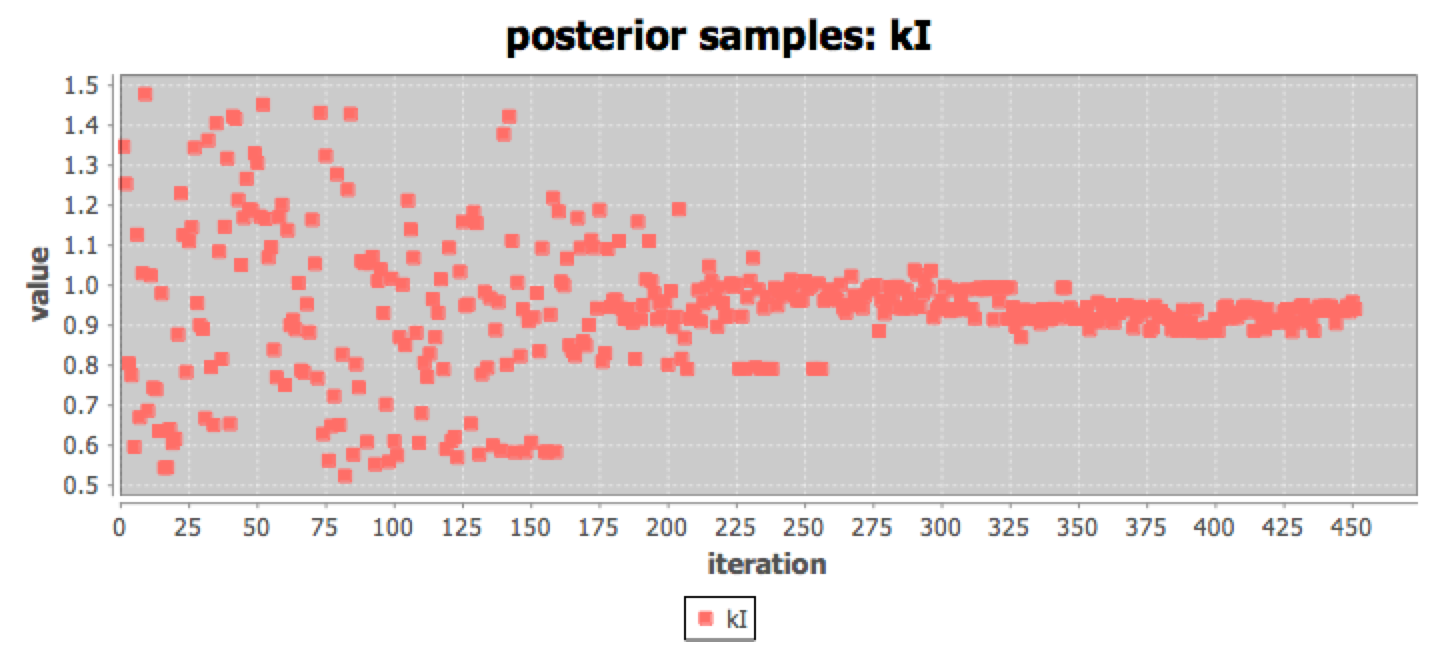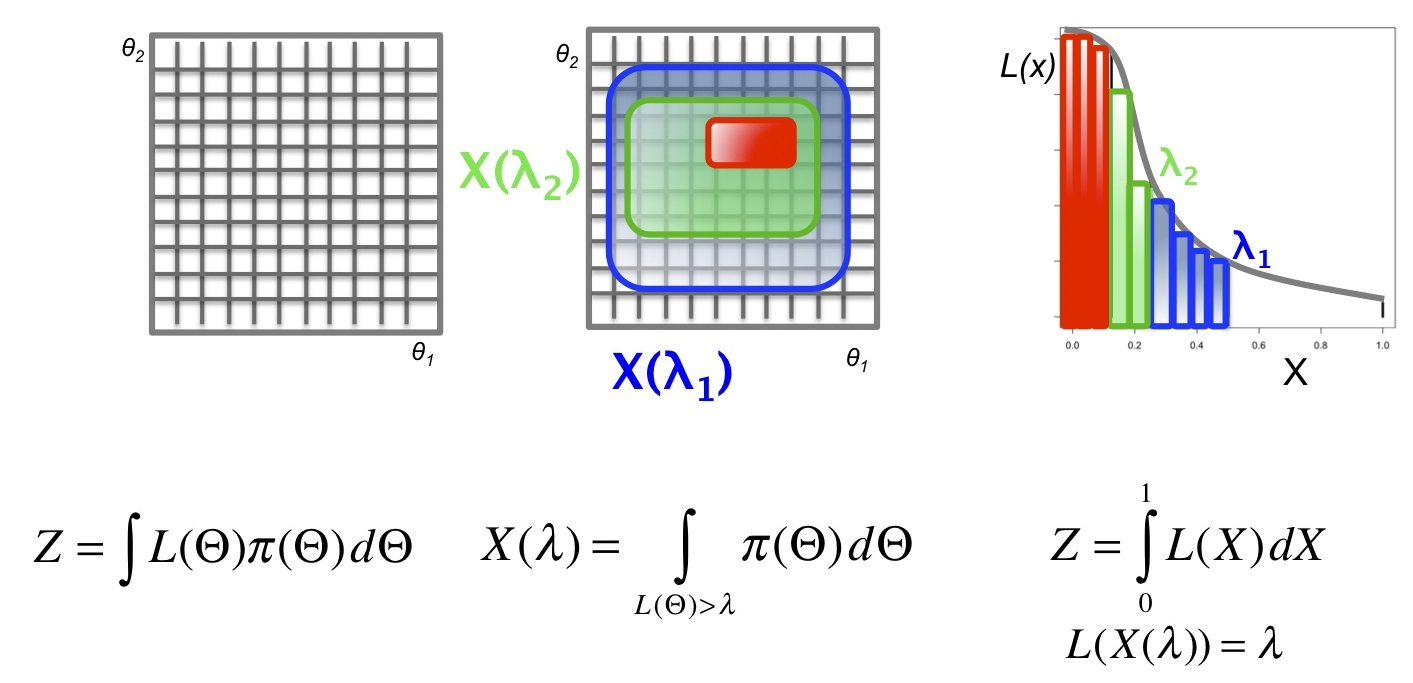## Parameter inference and model comparison in computational systems biology

Tools to infer parameters (i.e. to compute their mean and standard deviation) and compare models are being developed based around the nested sampling algorithm (Skilling, 2006) . The approach is readily applicable to any probabilistic modelling technique where a likelihood function can be defined. In this project, we focus on stochastic models of circadian rhythms, develop the code needed to run nested sampling reliably, and define likelihood functions for time-series data that can be computed efficiently.
This project is a collaboration between:

## Key results

Our methodology has been published. Using nested sampling, we demonstrate in an exemplar circadian model that the estimates of posterior parameter densities (as summarised by parameter means and standard deviations) are influenced predominately by the length of the time series, becoming more narrowly constrained as the number of circadian cycles considered increases. We also show the utility of the coefficient of variation for discriminating between highly-constrained and less-well constrained parameters:
Nested sampling for parameter inference in systems biology: application to an exemplar circadian model Stuart Aitken and Ozgur E Akman.
R code for nested sampling is available in the supplementary information.

## Dizzy Beats Java tool released

Dizzy Beats provides model simulators from the original Dizzy tool, and adds an optimiser and the nested sampling algorithm in an easy to use application. The Bayesian evidence, and parameter means and standard deviations are calculated, and diagnostic plots of posterior samples can be inspected. The results are saved for futher analysis. The Dizzy Beats tool makes our methodology more easily available to a wider range of users.Model selection answers the question: Given two or more models, and one or more data sets, which model structure (topology) explains the data best? Calculating the Bayesian evidence for a model is a quantitative approach to answering this question. The Bayesian evidence is the result of an integration over parameter values, rather than a point estimate of the goodness of fit for some specific combination of parameter values - which will typically only be guaranteed to be locally optimal, and therefore limit the comparisons that can be made between the models.

The integration of the likelihood function (L), multiplied by the prior (pi), over the parameter space gives the evidence Z. A model with n free parameters will require integration over an n dimensional space. (In contrast, maximum likelihood methods attempt to find the combination of parameters in n dimensional space that maximise L.) Nested sampling transforms the integral into one dimension: X. The new representation X is the enclosed prior mass.

The figure below illustrates the mapping from a 2-dimensional integration (left), through contours of enclosed prior mass corresponding to lambda-1 and lambda-2 (middle), to the sorted 1-dimensional plot of likelihood against X (right). The new likelihood function inverts X and the evidence integrates L over X.In this form, the integral must still be solved analytically. The next step is to sample from the prior (represented by the black circles in the diagram below), and, supposing we know how much of the prior mass is enclosed within the likelihood contours associated with each sample, the integral can be approximated by a sum. The upper and lower bounds on the integral can be obtained from the widths (delta Xi) and heights (L(Xi)) of the rectangular areas.The final element of the solution follows from the fact that if a set of N samples is constructed (initially, uniformly at random from the prior) and the worst is removed, the enclosed prior mass will shrink geometrically according to the distribution Beta(N,1). Nested sampling maintains a set of N samples - on each iteration the worst is removed, another sample is selected as the seed for generating a new sample (uniformly from the prior but with likelihood greater than that of the worst sample) and the new sample is added to the set. The average reduction in the enclosed prior will be 1/N and the error in the integral resulting from the distribution of shrinkages can be quantified.

The nested sampling algorithm is straightforward, but relies on being able to sample new points uniformly from the prior in a constrained and efficient manner. It is also necessary to implement a heuristic for termination. Likelihoods for time-series data are also required. These will be described in a forthcoming paper.

A number of use cases provide data and models that validate the computational methods. The most important of these comes from the Millar Lab where one, two and three loop models of circadian rhythms in Arabidopsis thaliana are being fitted to data obtained under varying experimental conditions. The majority of clock models that have been developed thus far, including those for Arabidopsis, are sets of coupled deterministic differential equations. However, stochastic versions of these models are becoming increasingly popular tools in computational circadian studies. Such models are considered to more accurately reflect the cellular environment in which the relatively small numbers of mRNAs and proteins comprising the clock yield significant stochastic fluctuations in expression levels; consideration of stochastic effects is thus necessary to fully quantify the molecular mechanisms controlling circadian timing.

Moreover, by being more representative of laboratory conditions, stochastic models can generate predictions that lie outside the scope of purely deterministic ones. For example, a recent stochastic model of the reduced Arabidopsis clock that is found in the unicellular alga Ostreococcus tauri gave insights into the optimal molecular species to use as an experimental phase maker in this system, as well as the minimum cellular population required to determine whether free-running oscillations occur at the single-cell level (Akman et al, 2010). Taken together, these considerations indicate that stochastic models are key to constructing viable likelihood functions when using Bayesian approaches to infer parameter values.

The model comparison functions are delivered to users by incorporating them into a new version of the popular stochastic simulation tool Dizzy (Ramsey et al, 2005) "Dizzy-Beat" [Bayesian evidence analysis tools].
The code will be made available open source via this SourceForge site:
https://sourceforge.net/p/bayesevidence/